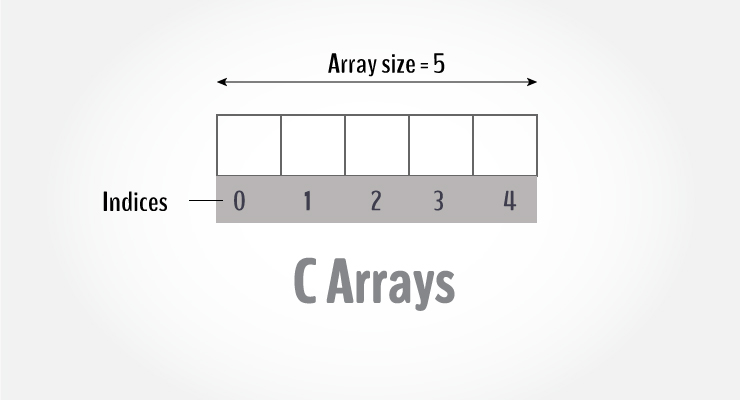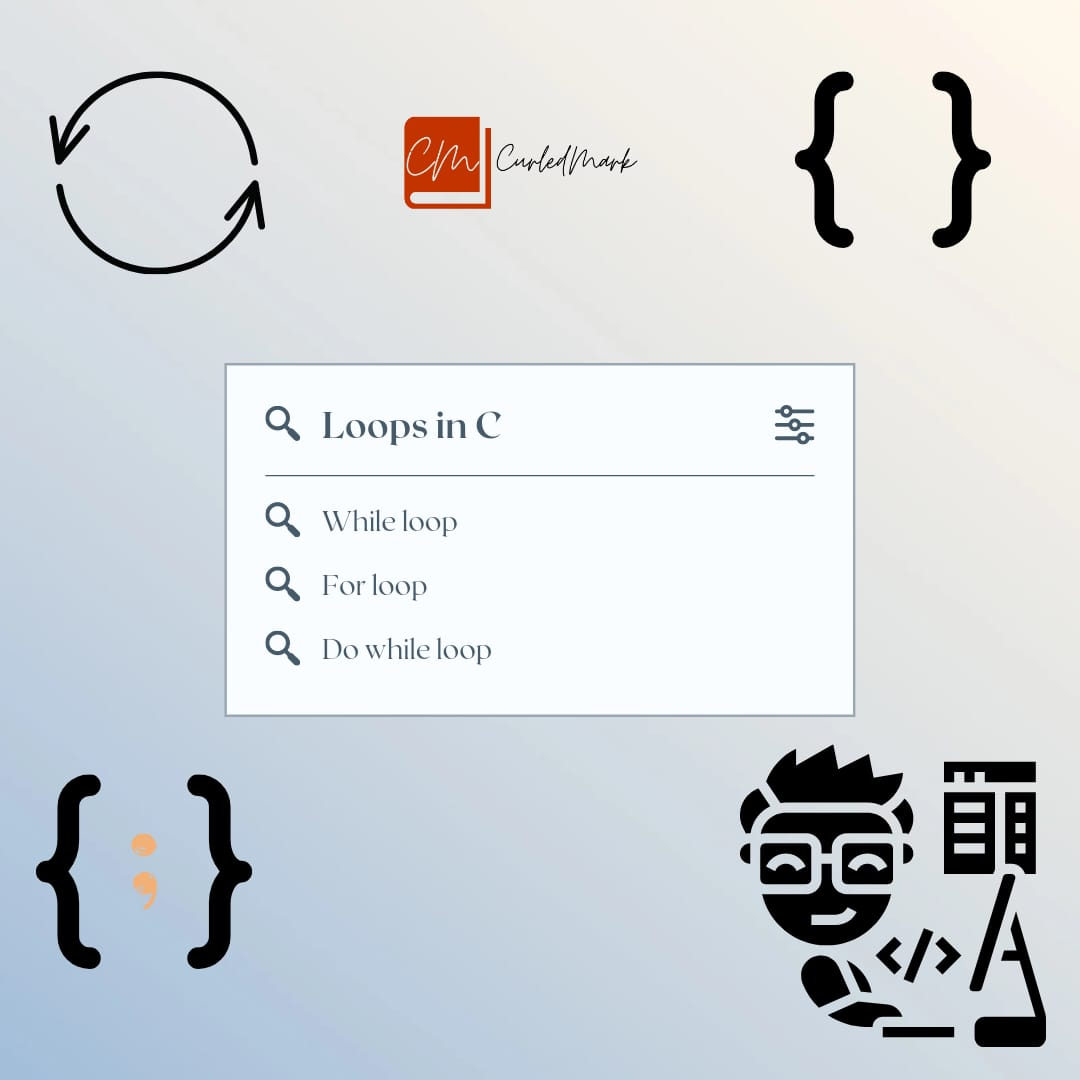September 25, 2022# Armstrong number and arrays in C Programming

## Armstrong number and arrays in C Programming

In this article, it is explained the Armstrong number program and the basic details of C Programming. In the next one you will get more details and programs based on C.

## Armstrong number

An Armstrong number is one whose sum of digits raised to the power three equals the number itself. 371, for example, is an Armstrong number because 3**3 + 7**3 + 1**3 = 371.

### Algorithm

• Find the number of digits (dc) = exp
• Separate each digit = base
• Find base to the power of exp as a result
• Result, k=k+result
• if k==x, then it is Armstrong number### Program

`#include<stdio.h>`

`int main()`

`{`

`int x,num,k,result,exp,base,dc,d,j;`

`Printf("Enter a number:");`

`Scanf("%d",&x);`

`if(x<0)`

`{`

`Printf("Not an armstrong number");`

`return 0;`

`}`

`num=x;`

`dc=1;`

` While(num>9)`

`{`

`dc++;`

`num=num/10;`

`}`

`k=0;exp=dc;`

`num=x;`

`While(num>0)`

`{`

`d=num%10;`

`base=d;`

`result=1;`

`j=1;`

`While(j<=exp)`

`{`

`result=result*base;`

`j++;`

`}`

`k+=result;`

`num/=10;`

`}`

`if(k==x)`

`{`

`Printf(%d is armstrong number\n,x");`

`}`

`else`

`{`

`Printf(%d is not an armstrong number",x);`

`}`

`return 0;`

`}`

## Arrays in C

An array is a collection of elements of the same type placed in contiguous memory locations that can be individually referenced by using an index to a unique identifier. Five values of type int can be declared as an array without having to declare five different variables.

To create an array, define the data type (like `int`) and specify the name of the array followed by square brackets [].

int x;

x is an array of 5 elements with indexes from 0 to 4.

Arrays are the derived data type in C programming language which can store the primitive type of data such as int, char, double, float, etc.

### Accept 10 numbers in an array. Compute and print the sum.

`#include<stdio.h>`

`int main()`

`{`

`int x,y,total;y=0;`

`While(y<=9)`

`{`

`Printf("Enter a number:");`

`Scanf("&d",&x[y]); `

`y++;`

`}`

`y=0;`

`total=0;`

`While(y<=9)`

`{`

`total = total+x[y];`

`y++;`

`Printf("Total is %d",total);`

` ``return 0;`

`} `

The Great Gatsby

The Forty Rules of Love Summary

The Norwegian Wood by Murakami Summary

Relentless Summary

The Da Vinci Code by Dan Brown Summary

The Book Of Five Rings Summary

The Blue Umbrella

How to Talk to Anyone by Leil Lowndes

Jonathan Livingston Seagull Book Summary

Your Brain on PORN Book Summary by Gary Wilson

THE IMMORTALS OF MELUHA By Amish Tripathi full summary

Eleven Minutes by Paulo Coelho Summary

Hyperfocus by Chris Bailey Summary

Black Beauty by Anna Sewell Summary

You Can Heal Your Life by Louise Hay Summary

ATTITUDE IS EVERYTHING BY JEFF KELLER SUMMARY

THE BIOGRAPHY OF SWAMI VIVEKANANDA

THE SHAWSHANK REDEMPTION EXPLAINED

Turtles All the Way Down by John Green Story Explained

Essentialism The Disciplined Pursuit of Less by Greg McKeown Summary

The One from the Stars by Keshav Aneel

#### You may have missed#### What is do-while loop in C Programming ? Loops